# Aquarium

The box-shaped aquarium is 40 cm high; the bottom has dimensions of 70 cm and 50 cm. Simon wanted to create an exciting environment for the fish, so he fixed three pillars to the bottom. They all have the shape of a cuboid with a square base. The base edge of each block is 10cm long. The heights of the blocks are 10cm, 20cm, 30cm. How many liters of water should be poured into the aquarium to reach a height of 25cm?

Result

V =  81.5 l

#### Solution:

$a = 70 \ cm = 70 / 10 \ dm = 7 \ dm \ \\ b = 50 \ cm = 50 / 10 \ dm = 5 \ dm \ \\ c = 25 \ cm = 25 / 10 \ dm = 2.5 \ dm \ \\ \ \\ V_{ 1 } = a \cdot \ b \cdot \ c = 7 \cdot \ 5 \cdot \ 2.5 = \dfrac{ 175 }{ 2 } = 87.5 \ dm^3 \ \\ \ \\ x = 10 \ cm = 10 / 10 \ dm = 1 \ dm \ \\ o = (10+20+30)/10 = 6 \ dm \ \\ \ \\ V_{ 2 } = x \cdot \ x \cdot \ o = 1 \cdot \ 1 \cdot \ 6 = 6 \ dm^3 \ \\ \ \\ V = V_{ 1 }-V_{ 2 } = 87.5-6 = \dfrac{ 163 }{ 2 } = 81.5 = 81.5 \ \text { l }$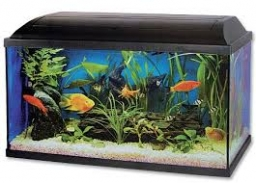Our examples were largely sent or created by pupils and students themselves. Therefore, we would be pleased if you could send us any errors you found, spelling mistakes, or rephasing the example. Thank you!

Leave us a comment of this math problem and its solution (i.e. if it is still somewhat unclear...):Be the first to comment!#### Following knowledge from mathematics are needed to solve this word math problem:

Do you know the volume and unit volume, and want to convert volume units?

## Next similar math problems:

1. The aquariumThe aquarium has a capacity of 18 liters. What is its height when the square bottom is 8 2/3 cm long?
2. Aquarium volumeThe aquarium has a cuboid shape and dimensions a = 0.3 m, b = 0.85 m, c =? , V = ?. What volume has a body, if after dipping into the aquarium water level rises by 28 mm?
3. AquariumAquarium is rectangular box with square base containing 76 liters of water. Length of base edge is 42 cm. To what height the water level goes?
4. The cuboidThe cuboid is filled to the brim with water. The external dimensions are 95 cm, 120 cm, and 60 cm. The thickness of all walls and the bottom is 5 cm. How many liters of water fit into the cuboid?
5. Swimming poolThe swimming pool has the shape of a block with dimensions of 70dm, 25m, 200cm. How many hl of water can fit into the pool?
6. DiggingA pit is dug in the shape of a cuboid with dimensions 10mX8mX3m. The earth taken out is spread evenly on a rectangular plot of land with dimensions 40m X 30m. What is the increase in the level of the plot ?
7. Concrete pillar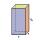How many m³ of concrete is needed for the construction of the pillar shape of a regular tetrahedral prism, when a = 60 cm and the height of the pillar is 2 meters?
8. HectolitersHow many hectoliters of water fits into cuboid tank with dimensions of a = 3.5 m b = 2.5 m c = 1.4 m?
9. Wood prismsHow many weight 25 prisms with dimensions 8x8x200 cm? 1 cubic meter of wood weighs 800 kg.
10. The shopThe shop has 3 hectoliters of water. How many liter bottles is it?
11. Cylindrical tank 2If a cylindrical tank with volume is used 12320cm raised to the power of 3 and base 28cm is used to store water. How many liters of water can it hold?
12. Rainwater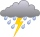The garden area of 800 square meters fell 3mm of rainwater. How many 10 liters of water can we water this garden equally?
13. Two cuboidsFind the volume of cuboidal box whose one edge is: a) 1.4m and b) 2.1dm
14. Percitipation - meteo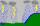Do you have any tip for calc percitipation on a lnd ? Example on 2 ha falls to 5 mm, how many cubic meters is it?
15. Truck of milk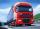How many hectoliters of ,,the box" milk fit in the truck, ake cargo size area is 2.8 m x 3 m x 17 m? A liter of milk in a box measuring 12 cm x 7 cm x 20 cm.
16. Water reservoirThe water tank has a cuboid with edges a= 1 m, b=2 m , c = 1 m. Calculate how many centimeters of water level falls, if we fill fifteen 12 liters cans.
17. Reservoir 3How many cubic feet of water is stored in the reservoir that has a length of 200 feet, a width of 100 feet, an overflow depth of 32 feet, and a current water level of 24 feet?